# Scalar weak maximum principle

(Redirected from Maximum principle)

## Definition

### Basic definition

Let$M$ be a differential manifold and$F$ be a differential operator that acts on functions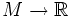$M \to \R$. Consider the flow equation associated with$f$, namely the equation for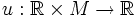$u:\R \times M \to \R$ given as: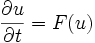$\frac{\partial u}{\partial t} = F(u)$

An initial value problem corresponding to this differential equation is a specification of$u(0,x)$ for each$x \in M$.

The differential operator$F$ is said to satisfy the scalar weak maximum principle if whenever$u$ is a solution for which there are constants$C_1$ and$C_2$ such that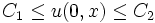$C_1 \le u(0,x) \le C_2$ for all$x \in M$, then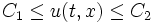$C_1 \le u(t,x) \le C_2$ for all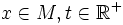$x \in M, t \in \R^+$.

In other words, any bounded set in which the range of$u(0,x)$ lies also contains the image of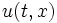$u(t,x)$ for all$t$.

### Definition in terms of trajectory properties

A differential operator$F$ is said to satisfy the scalar weak maximum principle if the trajectories of the corresponding flow equation are all bound-narrowing.

## Relation with other properties=

### One-sided maximum principles

• The flow equation is said to satisfy a one-sided scalar weak maximum principle if the trajectories of the flow equation are only min-increasing